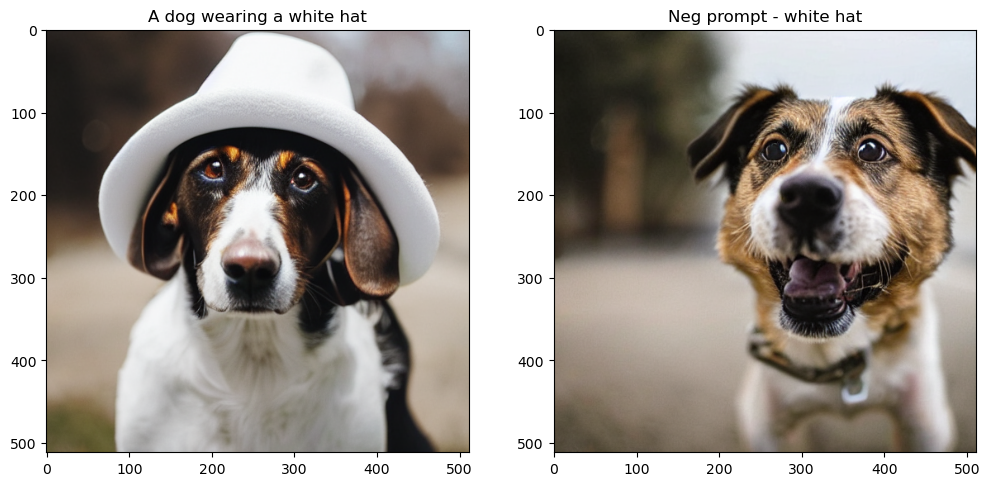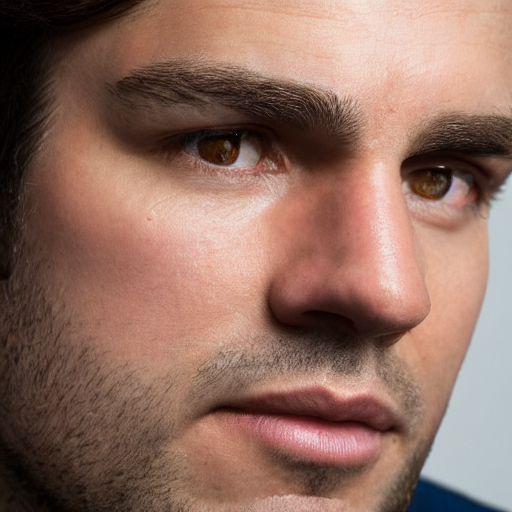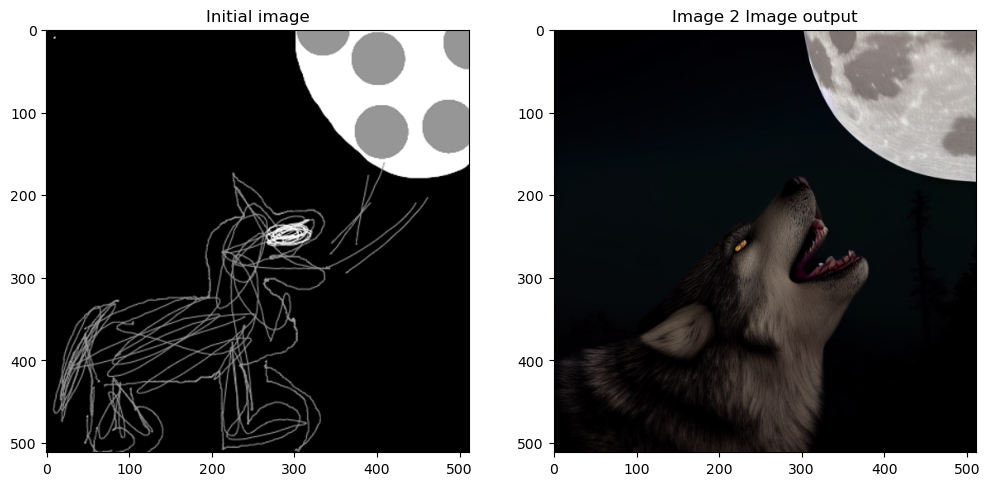# Stable diffusion using 🤗 Hugging Face - Variations of Stable Diffusion

Stable Diffusion
Author

Aayush Agrawal

Published

November 11, 2022

An introduction to negative prompting and image to image stable diffusion pipeline using 🤗 hugging face diffusers library.

This is my fourth post of the Stable diffusion series, if you haven’t checked out the previous ones, you can read it here -
1. Part 1 - Stable diffusion using 🤗 Hugging Face - Introduction.
2. Part 2 - Stable diffusion using 🤗 Hugging Face - Looking under the hood.
3. Part 3 - Stable diffusion using 🤗 Hugging Face - Putting everything together

In previous posts, I went over all the key components of Stable Diffusion and how to get a `prompt to image` pipeline working. In this post, I will show how to edit the `prompt to image` function to add additional functionality to our Stable diffusion pipeline i.e., `Negative prompting` and `Image to Image` pipeline. Hopefully, this will provide enough motivation to play around with this function and conduct your research.

## 1 Variation 1: Negative Prompt

### 1.1 What is negative prompting?

A negative prompt is an additional capability we can add to our model to tell the stable diffusion model what we don’t want to see in the generated image. This feature is popular to remove anything a user doesn’t want to see from the original generated image.

### 1.2 Understanding negative prompting through code

Let’s start by importing the required libraries and helper functions. All of this was already used and explained in the previous part 2 and part 3 of the series.

Code
``````import torch, logging

## disable warnings
logging.disable(logging.WARNING)

## Imaging  library
from PIL import Image
from torchvision import transforms as tfms

## Basic libraries
import numpy as np
from tqdm.auto import tqdm
import matplotlib.pyplot as plt
%matplotlib inline
from IPython.display import display
import shutil
import os

## For video display
from IPython.display import HTML
from base64 import b64encode

## Import the CLIP artifacts
from transformers import CLIPTextModel, CLIPTokenizer
from diffusers import AutoencoderKL, UNet2DConditionModel, LMSDiscreteScheduler

## Initiating tokenizer and encoder.
tokenizer = CLIPTokenizer.from_pretrained("openai/clip-vit-large-patch14", torch_dtype=torch.float16)
text_encoder = CLIPTextModel.from_pretrained("openai/clip-vit-large-patch14", torch_dtype=torch.float16).to("cuda")

## Initiating the VAE
vae = AutoencoderKL.from_pretrained("CompVis/stable-diffusion-v1-4", subfolder="vae", torch_dtype=torch.float16).to("cuda")

## Initializing a scheduler and Setting number of sampling steps
scheduler = LMSDiscreteScheduler(beta_start=0.00085, beta_end=0.012, beta_schedule="scaled_linear", num_train_timesteps=1000)
scheduler.set_timesteps(50)

## Initializing the U-Net model
unet = UNet2DConditionModel.from_pretrained("CompVis/stable-diffusion-v1-4", subfolder="unet", torch_dtype=torch.float16).to("cuda")

## Helper functions
'''
Function to load images from a defined path
'''
return Image.open(p).convert('RGB').resize((512,512))

def pil_to_latents(image):
'''
Function to convert image to latents
'''
init_image = tfms.ToTensor()(image).unsqueeze(0) * 2.0 - 1.0
init_image = init_image.to(device="cuda", dtype=torch.float16)
init_latent_dist = vae.encode(init_image).latent_dist.sample() * 0.18215
return init_latent_dist

def latents_to_pil(latents):
'''
Function to convert latents to images
'''
latents = (1 / 0.18215) * latents
image = vae.decode(latents).sample
image = (image / 2 + 0.5).clamp(0, 1)
image = image.detach().cpu().permute(0, 2, 3, 1).numpy()
images = (image * 255).round().astype("uint8")
pil_images = [Image.fromarray(image) for image in images]
return pil_images

def text_enc(prompts, maxlen=None):
'''
A function to take a texual promt and convert it into embeddings
'''
if maxlen is None: maxlen = tokenizer.model_max_length
inp = tokenizer(prompts, padding="max_length", max_length=maxlen, truncation=True, return_tensors="pt")
return text_encoder(inp.input_ids.to("cuda")).half()``````

Now we are going to change the `prompt_2_img` function from part 3 by passing an additional function `neg_prompts`. The way negative prompt works is by using user-specified text instead of an empty string for unconditional embedding(`uncond`) when doing sampling.

So let’s make this change and update our `prompt_2_img` function.

``````def prompt_2_img(prompts, neg_prompts=None, g=7.5, seed=100, steps=70, dim=512, save_int=False):
"""
Diffusion process to convert prompt to image
"""

# Defining batch size
bs = len(prompts)

# Converting textual prompts to embedding
text = text_enc(prompts)

# Adding an unconditional prompt , helps in the generation process
if not neg_prompts: uncond =  text_enc([""] * bs, text.shape)
else: uncond =  text_enc(neg_prompts, text.shape)
emb = torch.cat([uncond, text])

# Setting the seed
if seed: torch.manual_seed(seed)

# Initiating random noise
latents = torch.randn((bs, unet.in_channels, dim//8, dim//8))

# Setting number of steps in scheduler
scheduler.set_timesteps(steps)

# Adding noise to the latents
latents = latents.to("cuda").half() * scheduler.init_noise_sigma

# Iterating through defined steps
for i,ts in enumerate(tqdm(scheduler.timesteps)):
# We need to scale the i/p latents to match the variance
inp = scheduler.scale_model_input(torch.cat([latents] * 2), ts)

# Predicting noise residual using U-Net
with torch.no_grad(): u,t = unet(inp, ts, encoder_hidden_states=emb).sample.chunk(2)

# Performing Guidance
pred = u + g*(t-u)

# Conditioning  the latents
latents = scheduler.step(pred, ts, latents).prev_sample

# Saving intermediate images
if save_int:
if not os.path.exists(f'./steps'): os.mkdir(f'./steps')
latents_to_pil(latents).save(f'steps/{i:04}.jpeg')

# Returning the latent representation to output an image of 3x512x512
return latents_to_pil(latents)``````

Let’s see if the function works as intended.

``````## Image without neg prompt
images = [None, None]
images = prompt_2_img(prompts = ["A dog wearing a white hat"], neg_prompts=[""],steps=50, save_int=False)
images = prompt_2_img(prompts = ["A dog wearing a white hat"], neg_prompts=["White hat"],steps=50, save_int=False)

## Plotting side by side
fig, axs = plt.subplots(1, 2, figsize=(12, 6))
for c, img in enumerate(images):
axs[c].imshow(img)
if c == 0 : axs[c].set_title(f"A dog wearing a white hat")
else: axs[c].set_title(f"Neg prompt - white hat")``````As we can see it can be a really handy feature to fine-tune the image to your liking. You can also use it to generate a pretty realistic face by being really descriptive as this Reddit post. Let’s try it -

``````prompt = ['Close-up photography of the face of a 30 years old man with brown eyes, (by Alyssa Monks:1.1), by Joseph Lorusso, by Lilia Alvarado, beautiful lighting, sharp focus, 8k, high res, (pores:0.1), (sweaty:0.8), Masterpiece, Nikon Z9, Award - winning photograph']
neg_prompt = ['lowres, signs, memes, labels, text, food, text, error, mutant, cropped, worst quality, low quality, normal quality, jpeg artifacts, signature, watermark, username, blurry, made by children, caricature, ugly, boring, sketch, lacklustre, repetitive, cropped, (long neck), facebook, youtube, body horror, out of frame, mutilated, tiled, frame, border, porcelain skin, doll like, doll']
images = prompt_2_img(prompts = prompt, neg_prompts=neg_prompt, steps=50, save_int=False)
images``````Pretty neat! I hope this gives you some ideas on how to get going with your own variations of stable diffusion. Now let’s look at another variation of Stable diffusion.

## 2 Variation 2: Image to Image pipeline

### 2.1 What is an image to image pipeline?

As seen above, `prompt_2_img` functions start generating an image from random gaussian noise, but what if we feed an initial seed image to guide the diffusion process? This is exactly how the image to image pipeline works. Instead of purely relying on text conditioning for the output image, we can use an initial seed image mix it with some noise (which can be guided by a `strength` parameter), and then run the diffusion loop.

### 2.2 Understanding image to image prompting through code

Now we are going to change the `prompt_2_img` function defined above. We will introduce two more parameters to our `prompt_2_img_i2i` function -
1. `init_img`: Which is going to be the `Image` object containing the seed image
2. `strength`: This parameter will take a value between 0 and 1. The higher the value less the final image is going to look similar to the seed image.

``````def prompt_2_img_i2i(prompts, init_img, neg_prompts=None, g=7.5, seed=100, strength =0.8, steps=50, dim=512, save_int=False):
"""
Diffusion process to convert prompt to image
"""
# Converting textual prompts to embedding
text = text_enc(prompt)

# Adding an unconditional prompt , helps in the generation process
if not neg_prompts: uncond =  text_enc([""], text.shape)
else: uncond =  text_enc(neg_prompt, text.shape)
emb = torch.cat([uncond, text])

# Setting the seed
if seed: torch.manual_seed(seed)

# Setting number of steps in scheduler
scheduler.set_timesteps(steps)

# Convert the seed image to latent
init_latents = pil_to_latents(init_img)

# Figuring initial time step based on strength
init_timestep = int(steps * strength)
timesteps = scheduler.timesteps[-init_timestep]
timesteps = torch.tensor([timesteps], device="cuda")

# Adding noise to the latents
noise = torch.randn(init_latents.shape, generator=None, device="cuda", dtype=init_latents.dtype)
latents = init_latents

# Computing the timestep to start the diffusion loop
t_start = max(steps - init_timestep, 0)
timesteps = scheduler.timesteps[t_start:].to("cuda")

# Iterating through defined steps
for i,ts in enumerate(tqdm(timesteps)):
# We need to scale the i/p latents to match the variance
inp = scheduler.scale_model_input(torch.cat([latents] * 2), ts)

# Predicting noise residual using U-Net
with torch.no_grad(): u,t = unet(inp, ts, encoder_hidden_states=emb).sample.chunk(2)

# Performing Guidance
pred = u + g*(t-u)

# Conditioning  the latents
latents = scheduler.step(pred, ts, latents).prev_sample

# Saving intermediate images
if save_int:
if not os.path.exists(f'./steps'):
os.mkdir(f'./steps')
latents_to_pil(latents).save(f'steps/{i:04}.jpeg')

# Returning the latent representation to output an image of 3x512x512
return latents_to_pil(latents)``````

Instead of using random noise, you will notice we use the `strength` parameter to figure out how much noise to add and also the number of steps to run the diffusion loop for. The amount of noise is calculated by multiplying strength(default = 0.8) with steps (default = 50) which is the 10th (50 - 50 * 0.8) step and running the diffusion loop for 40(50*0.8) remaining steps. Let’s load an initial image and pass it through the `prompt_2_img_i2i` function.

``````p = FastDownload().download('https://s3.amazonaws.com/moonup/production/uploads/1664665907257-noauth.png')
image = Image.open(p).convert('RGB').resize((512,512))
prompt = ["Wolf howling at the moon, photorealistic 4K"]
images = prompt_2_img_i2i(prompts = prompt, init_img = image)``````
``````/home/aayush/miniconda3/envs/fastai/lib/python3.9/site-packages/diffusers/schedulers/scheduling_lms_discrete.py:146: IntegrationWarning: The maximum number of subdivisions (50) has been achieved.
If increasing the limit yields no improvement it is advised to analyze
the integrand in order to determine the difficulties.  If the position of a
local difficulty can be determined (singularity, discontinuity) one will
probably gain from splitting up the interval and calling the integrator
on the subranges.  Perhaps a special-purpose integrator should be used.
integrated_coeff = integrate.quad(lms_derivative, self.sigmas[t], self.sigmas[t + 1], epsrel=1e-4)``````
Code
``````## Plotting side by side
fig, axs = plt.subplots(1, 2, figsize=(12, 6))
for c, img in enumerate([image, images]):
axs[c].imshow(img)
if c == 0 : axs[c].set_title(f"Initial image")
else: axs[c].set_title(f"Image 2 Image output")``````We can see our `prompt_2_img_i2i` function creates a pretty epic image from the initial sketch provided.

## 3 Conclusion

I hope this gives a good overview of how to tweak the `prompt_2_img` function to add additional capabilities to your stable diffusion loop. The understanding of this lower-level function is useful for trying your own idea to improve stable diffusion or implement new papers which I might cover in my next post.

I hope you enjoyed reading it, and feel free to use my code and try it out for generating your images. Also, if there is any feedback on the code or just the blog post, feel free to reach out on LinkedIn or email me at aayushmnit@gmail.com.